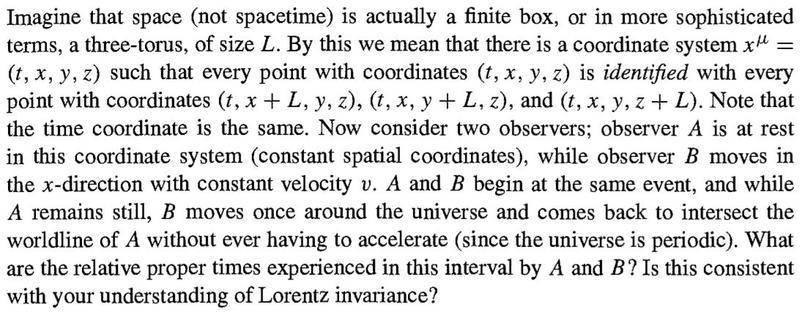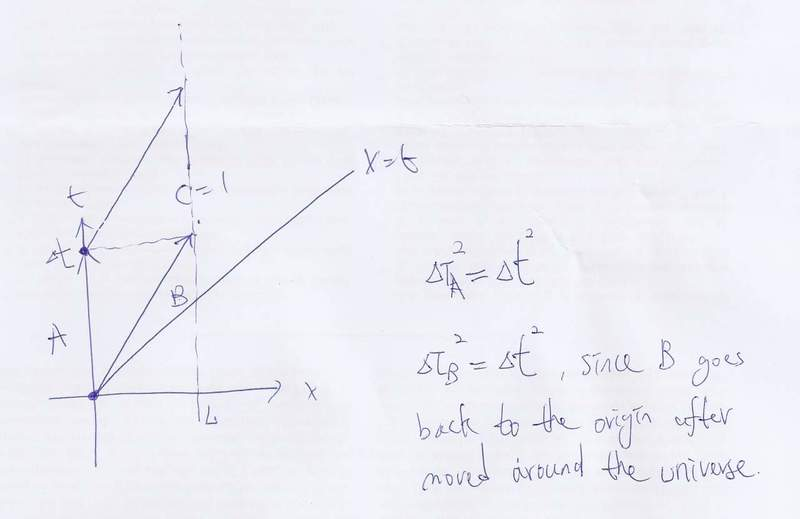# Space as a 3 torus

## Homework Statement## Homework Equations

spacetime interval ##Δs^2=-Δt^2+Δx^2+Δy^2+Δz^2##

## The Attempt at a SolutionWe know that the straight path in spacetime diagram is the one with maximum proper time, however we get the same value after going around the universe and this is not consistent of my understanding of Lorentz invariance.

I just want to know if my understanding is right or not.
Any comment would be appreciated,
Regards.

I don't understand your solution. From A's perspective, the clock of B runs slower so surely B ages more slowly than A.

Hello clamtrox,

Sorry for unclear solution.
What I thought is that, after a revolution from the universe, B travels back to ##x=0## point at the same time as A since ##x\sim x+L##.
Hence the proper time measured by B is the same as A, which contradicts my understanding of Lorentz invariance.

Regards.

What I thought is that, after a revolution from the universe, B travels back to ##x=0## point at the same time as A since ##x\sim x+L##.

Which reference frame are you using to compute the proper times? Can you show the calculation, as there's something wrong with it.

For the observer A, ##\Delta s_A^2 = -\Delta t^2 ##.
For the observer B, ##\Delta s_B^2 = -\Delta t^2 ##, since ##x=0\sim x=L##.

mfb
Mentor
You can identify the point in space, but not in time. You cannot just assume that the proper times are the same because they meet in the same point in space again.

What confused me is that is the coordinate time of B after travelling around the universe not the same as that of A?

mfb
Mentor
Coordinate time in which reference frame? For any observer, "meeting" means they have the same coordinate time. But this does not mean that they have to have the same proper time.

My calculations depend on the formula ##\Delta \tau^2 =- \Delta s^2 =\Delta t^2 -\Delta x^2##.
If the coordinate times are the same, I don't know where's the fault.

mfb
Mentor
This formula works in a regular space only.

Just because you identify the points x and x+L, that doesn't mean the distance you've travelled when you go around the universe is zero. In one case, you have $\Delta \tau^2 = \Delta t^2$, in the other $\Delta \tau^2 = \Delta t^2 - L^2$.